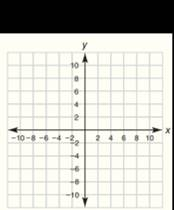Chapter 10.CT, Problem 5CT### Elementary Geometry for College St...

6th Edition
Daniel C. Alexander + 1 other
ISBN: 9781285195698

#### Solutions

Chapter
Section### Elementary Geometry for College St...

6th Edition
Daniel C. Alexander + 1 other
ISBN: 9781285195698
Textbook Problem
1 views

# Complete the following table of x and y-coordinates of points on the graph of the equation 2 x + 3 y = 12 . x 0 3 9 y 4To determine

Complete the following table by using the equation 2x+3y=12.

Explanation

Substitute the given values in the equation thus find the unknown values.

Substitute x=0 in the given equation 2x+3y=12,

20+3y=12

On simplifying,

3y=12

The coefficient of y is 3, thus divide both sides by 3.

3y3=123

y=4

Thus the first point is 0, 4.

Substitute x=3 in the given equation 2x+3y=12,

23+3y=12

On simplifying,

6+3y=12

3y=12-6

3y=6

The coefficient of y is 3, thus divide both sides by 3.

3y3=63

y=2

Thus the second point is 3, 2

### Still sussing out bartleby?

Check out a sample textbook solution.

See a sample solution

#### The Solution to Your Study Problems

Bartleby provides explanations to thousands of textbook problems written by our experts, many with advanced degrees!

Get Started

#### Find more solutions based on key concepts